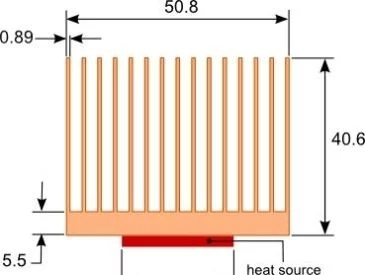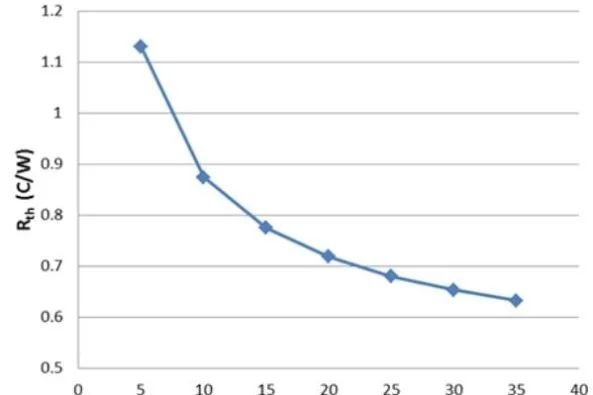# When the heat sinks cannot meet the cooling and heat dissipation requirements

2020-07-28

If the basic factors that will affect the performance of the heat sinks are not understood, the heat sink selected may not be sufficient to reduce the heat dissipation requirements of the cooling equipment. This will lead to loop iterations of design and test tasks, and ultimately lead to the development process of error-coded products.

Generally, when evaluating the performance of a heat sink, there are the following 3 common mistakes. .

1. Use the thermal resistance provided by the product manufacturer to evaluate the performance of the heat sink

As shown in Equation 1, the housing temperature Tc of the device can be determined by multiplying the thermal resistance Rth of the heat sink by the power dissipation Q of the device to be cooled, plus the ambient temperature Tamb. The typical method of selecting a heat sink is to first use Equation 1 to calculate the required heat sink thermal resistance, and then select a heat sink with a thermal resistance less than or equal to the calculated value.

(1) The thermal resistance provided by the heat sink manufacturer is generally measured through a square heat source heat sink, usually a square heat source (fixed heat loss) with a size of 25.4 mm x 25.4 mm is connected to the center of the bottom surface of the heat sink substrate, and then By measuring the temperature difference, the thermal resistance of the heat sink can be calculated by formula 1.

If the thermal resistance value measured by this method is used to select a heat sink, it will often happen that the selected heat sink does not meet the heat dissipation requirements, because the thermal resistance of the heat sink is not a constant. It changes with respect to the size of the base surface of the heat sink.

If the heat source you use is significantly smaller than the heat source used by the manufacturer when testing the actual heat sink, the thermal resistance value may far exceed the manufacturer's test value. This is caused by heat diffusion and penetration when flowing from the heat source area to the top surface of the heat sink.

Relative to the substrate area of the heat sink, the smaller the area of the heat source, the expansion of the heat spread of the heat sink, and the total thermal resistance of the heat sink will change. In the case of forced convection cooling, the influence of convection diffusion on the heat sink is the most significant.

Figure 1 Schematic diagram of the heat sinks geometry modelFigure 2 The influence of heat source size on the thermal resistance of the heat sinksFigure 2 shows the relationship between the size of the heat source and the thermal resistance of the heat sink under forced convection cooling. The dimensions of the heat sink are shown in Figure 1. The length of the heat sink is 76.2 mm, and the length and width of the heat source are the same and determined by Ls. The flow rate is 5 CFM, the heat emitted by the heat source is 59 W, and the ambient temperature is 30°C.

The thermal resistance of the heat sinks provided by the heat sink manufacturer is usually for a heat sink with a length of 76.2 mm. It should be noted that when the heat sink profile is the same, the thermal resistance of the heat sink does not increase linearly with the length (that is, the depth of the heat sink). As the length increases, due to the overlap of the ratio of the area of the heat source to the area of the bottom of the heat sink, diffusion also increases. The heat transfer gains brought about by such partial increase are replaced by the increase of diffusion decomposition. As the length of the heat sink increases, the heat transfer efficiency of the heat sink during convection will also decrease.

Chat Online 编辑模式下无法使用
Chat Online inputting...